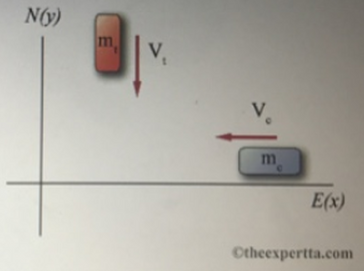# Problem: A car with mass mc = 1353 kg is travelling west through an intersection at a magnitude of velocity of vc = 6.5 m/s when a track of mass mt= 1647 kg travelling south at vt = 15 A m/s fails to yield and collides with the car. The vehicles become stuck together and slide on the asphalt, which has a coefficient of friction of μk = 0.5.Part (a) Write an expression for the veolocity of the system after the collision, in terms of the variables given in the problem statement and the unit vectors i and j. Part(b) How far, in meters, will the vehicles slide after the collision?

###### FREE Expert Solution

Part (a)

From the conservation of momentum:

mcvc + mtvt = (mc + mt)vf

vf = (mcvc + mtvt)/(mc + mt)

79% (432 ratings)###### Problem Details

A car with mass mc = 1353 kg is travelling west through an intersection at a magnitude of velocity of vc = 6.5 m/s when a track of mass mt= 1647 kg travelling south at vt = 15 A m/s fails to yield and collides with the car. The vehicles become stuck together and slide on the asphalt, which has a coefficient of friction of μk = 0.5.Part (a) Write an expression for the veolocity of the system after the collision, in terms of the variables given in the problem statement and the unit vectors i and j.

Part(b) How far, in meters, will the vehicles slide after the collision?